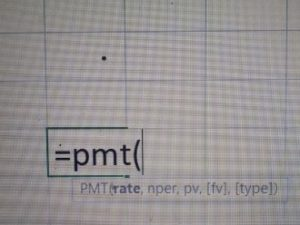# EMI of a Loan- How to Calculate it? ( Telugu)

1) EMI of a Loan- How to Calculate it? ( Telugu)

For Calculating it for a loan, you need three parameters.

1. Loan amount required.

2. No. of months of the repayment period

3. The Interest rate on loans.

If you know all the above parameters you can easily calculate the “EMI” of a loan.

There is a simple way to calculate “equated monthly installment” of loans in Micro soft Excel.

If you type =pmt( then in Excel formulae will open like this.By seeing the formulae above you can enter the values and then click the “Enter” button on the keyboard. Then you will get the “E.M.I” of loans that you are planning.